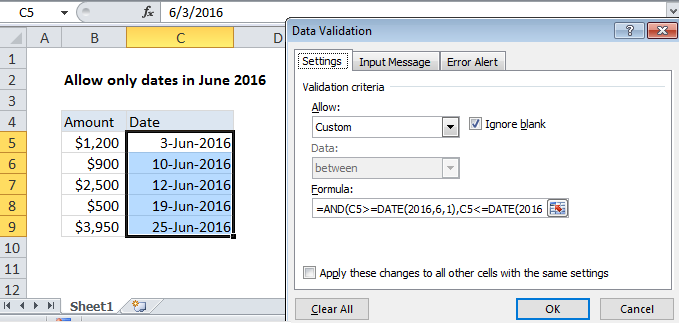## Excel Office

Excel How Tos, Tutorials, Tips & Tricks, Shortcuts

# Set criteria to accept only dates in a particular period

Excel has several built-in data validation rules for dates. This page explains how to create a your own validation rule based on a custom formula in case you want or need more control and flexibility.

## Formula

`=AND(A1>=date1),A1<=date2)`

##Explanation

To allow a user to enter only dates between two dates, you can use data validation with a custom formula based on the AND function.

Also See:   Highlight values between in Excel

In the example shown, the data validation applied to C5:C9 is:

`=AND(C5>=DATE(2016,6,1),C5<=DATE(2016,6,30))`

### How this formula works

Data validation rules are triggered when a user adds or changes a cell value.

The AND function takes multiple arguments (logicals) and returns TRUE only when all arguments return TRUE. The DATE function creates a proper Excel date with given year, month, and day values.

Also See:   Excel Data validation require unique number

Because we want to allow only dates in the month of June 2016, we give AND with two logicals.

The first logical tests that input to C5 is greater than or equal to June 1, 2016:

`C5>=DATE(2016,6,1)`

The second logical tests that input to C5 is less than or equal to June 30, 2016:

`C5<=DATE(2016,6,30)`

If both conditions are TRUE, the AND function returns TRUE and input passes validation. If either condition is FALSE, AND returns FALSE and input fails data validation.

Also See:   Find duplicate values in two columns in Excel